# CKM formalismis a 3x3 unitary matrix which originates from the misalignment in flavour space of the up and down components of the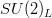quark doublet of the Standard Model (SM). In the quark mass eigenstate basis, the CKM matrix appears in the SM charged-current interaction Lagrangian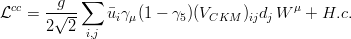where the quark fields areand, while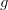is the weak coupling constant andis the field which creates the vector boson. The CKM matrix elements are the only flavour-non-diagonal and CP-violating couplings present in the SM. In general, the CKM matrix can be parametrized using three rotation angles and one phase. The parametrization is however not unique. The standard parametrization, advocated by the PDG, usesin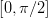andindefined so thatThe relations induced by the unitarity of the CKM matrix include six "triangular" relations, among whichis referred to as the Unitarity Triangle (UT). It can be rewritten as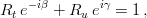with,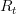and,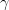are the non-trivial sides and angles of the normalized UT. The third side is the unit vector and the third angle is given by. The UT is determined by one complex numbernamely by the coordinatesin the complex plane of its only non-trivial apex (the others being (0,0) and (1,0)). Several experimental constraints can be conveniently represented on this plane and used to determine the UT, as shown in section Fit Results. We start extracting the CKM parameters from the measurements ofandusingThe sign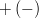in the formula forcorresponds to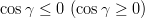. Additional constraints, discussed in section Constraints, are then applied using the method described in section Statistical Method. Fit Results are also given in the popular Wolfenstein parametrization which allows for a transparent expansion of the CKM matrix in terms of the sine of the small Cabibbo angle. The Wolfenstain parameters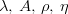are defined by the following equations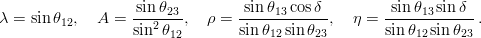The CKM matrix can be expanded asThe exact and expanded relations between the UT apex coordinatesand the Wolfenstein parameters are given by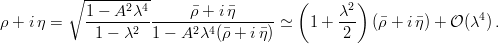At the lowest order in,and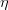coincide withand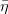.

Ideas, requests, problems regarding this web site? Send feedback